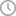# No, PSLE aggregate score is not out of 300

It is inaccurate to compare scores between cohorts across years.October 02, 10:30 AM

Singaporeans are probably familiar with the idea of PSLE T-scores.

But there are many common misconceptions about it.

## Calculating the T-score

The PSLE aggregate score is the sum of the T-score of all four subjects.

According to the Singapore Examinations and Assessment Board (SEAB), this is the formula for how the T-score is calculated:

x is the student’s raw score for the subject, m is the cohort’s average raw score for the subject, and S is the standard deviation (SD).

The standard deviation is a statistical measure for the amount of spread of the results from the average. The lower the standard deviation and cohort average, the higher the T-score for that subject.

For illustration, check out this worked example by Polymath Learning Centre:

## T-score not out of a possible maximum of 300

A student who gets full marks for all subjects may not get an aggregate score of 300.

This is because the T-score has to take into account other variables, such as the SD and cohort average.

Take the SD and cohort average for all the subjects in a particular year to be 20 and 60 respectively.

If a student got full marks for all subjects in that year, the T-score for each subject would be:

50+ 10(100-60)/20 = 70

And his/her PSLE aggregate score would be 4 x 70 = 280.

## Cannot be compared between cohorts

Based on the formula for calculating T-scores, it is also rather difficult to compare similar scores of two students from different cohorts.

A student with the same raw scores for each subject could have gotten a very different PSLE aggregate score in a different year.

This is because the T-scores are affected by the standard deviation and cohort average that is specific to that cohort of students taking the exam.

[related_story]

For illustration, take a look at these two tables from Road to PSLE:

Now you know.

Top photo via.

### Content that keeps Mothership.sg going

?
What was the last thing you used this place for? (Somebody said paktor, meet boys?) This is what you can do these days!

?
All the restaurants you ever wanted to try on a massive discount! Did somebody say 'Pince and Pints'?

?
Have a little money but can't help being kinda lazy? You can still invest using this.

?
This was not part of CIP.